# ICSE Class 8 Maths Selina Solutions Chapter 9 Simple and Compound Interest

Simple and compound Interest are mostly used in banking. You must have seen statements like “one year interest for a fixed deposit in the bank @7% per annum”. Do you understand the meaning of this? Well, it means if a person does the fixed deposit of some amount of money for 1 year then he/she will get 7% interest on it. Now, you must be wondering what this interest is? The Interest is the extra money paid by banks or post offices on the money that the user had deposited earlier. Chapter 9 of ICSE class 8 completely deals with the topics of Simple and Compound Interest. Here, you will learn how to calculate the difference between simple and compound interest, the formula for compound interest through patterns and questions related to them.

You must solve all the exercise problems in this chapter. In annual exams, you will definitely find some of the questions based on this topic. Moreover, this chapter will also improve your quantitative aptitude questions solving skills. Now, in most of the competitive examination quantitative aptitude is asked. So, this chapter will benefit you there also. To download the Simple and Compound Interest Selina solutions pdf click on the link below;

## Download ICSE Class 8 Maths Selina Solutions PDF for Chapter 9:-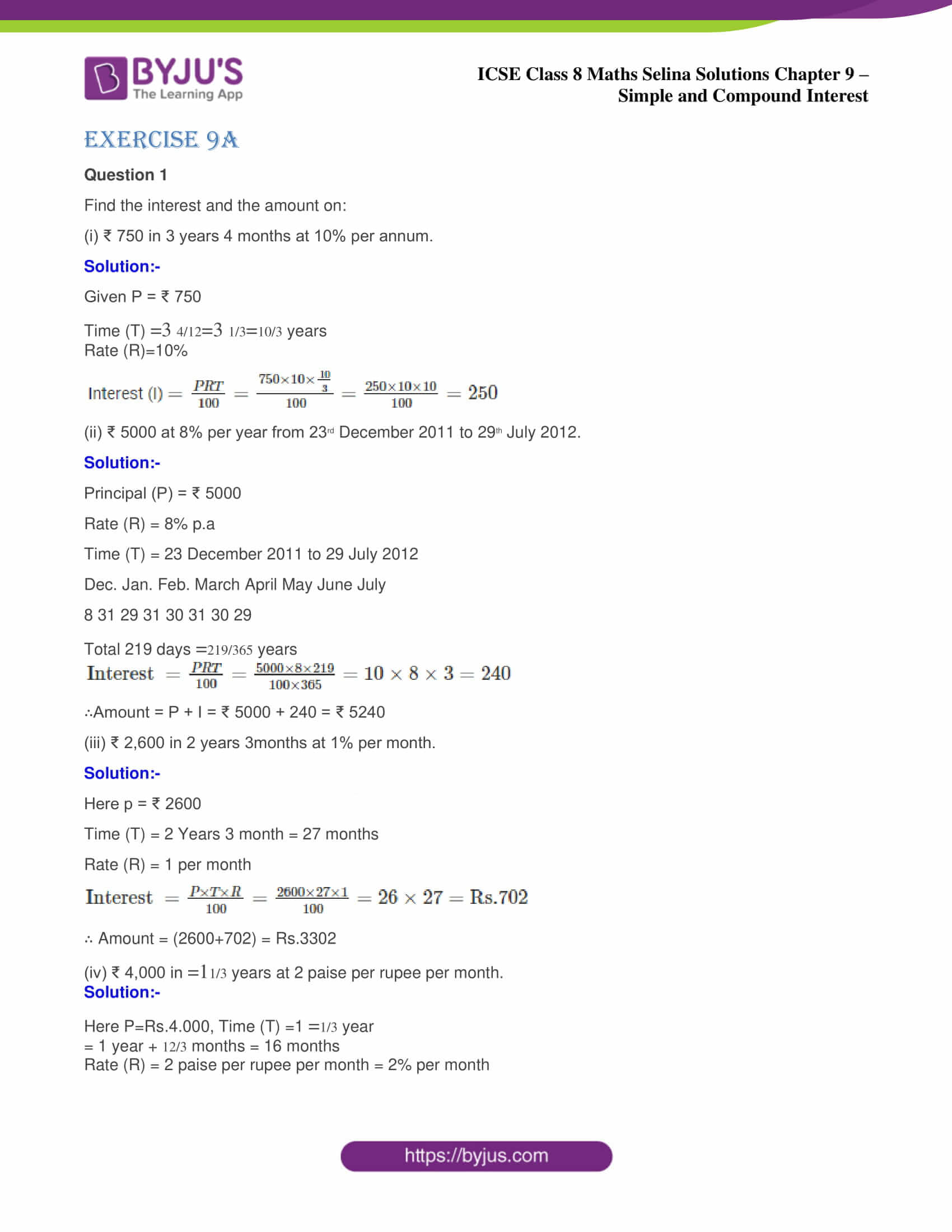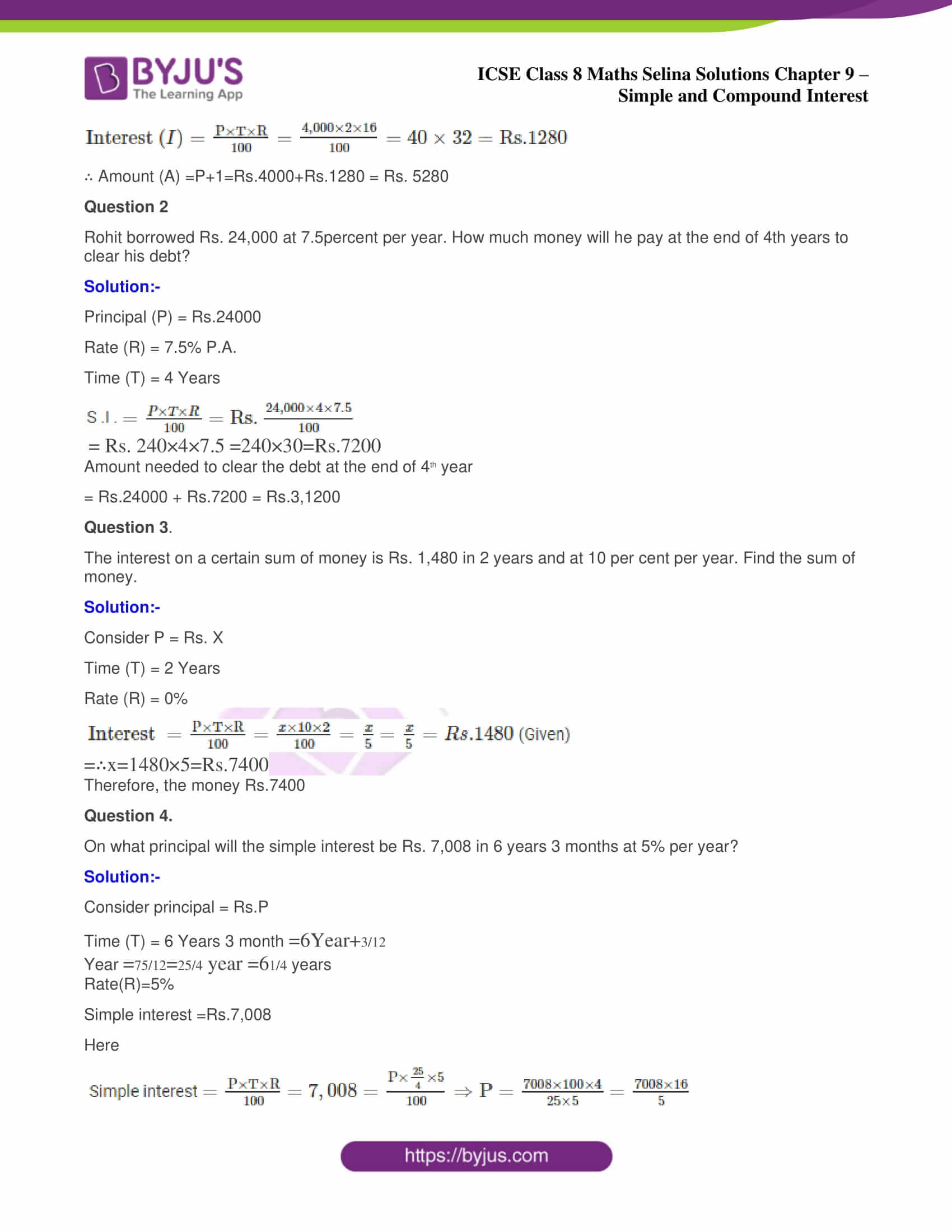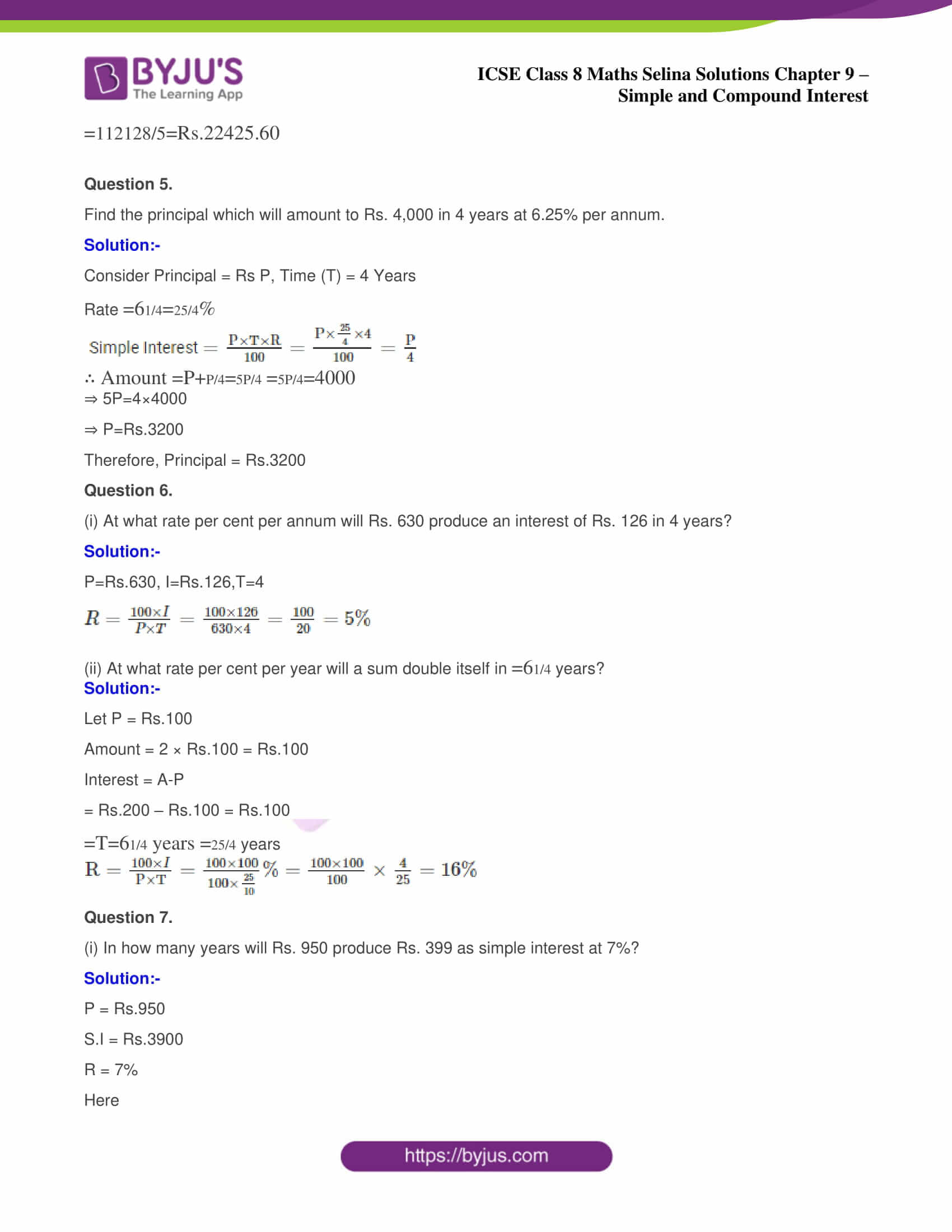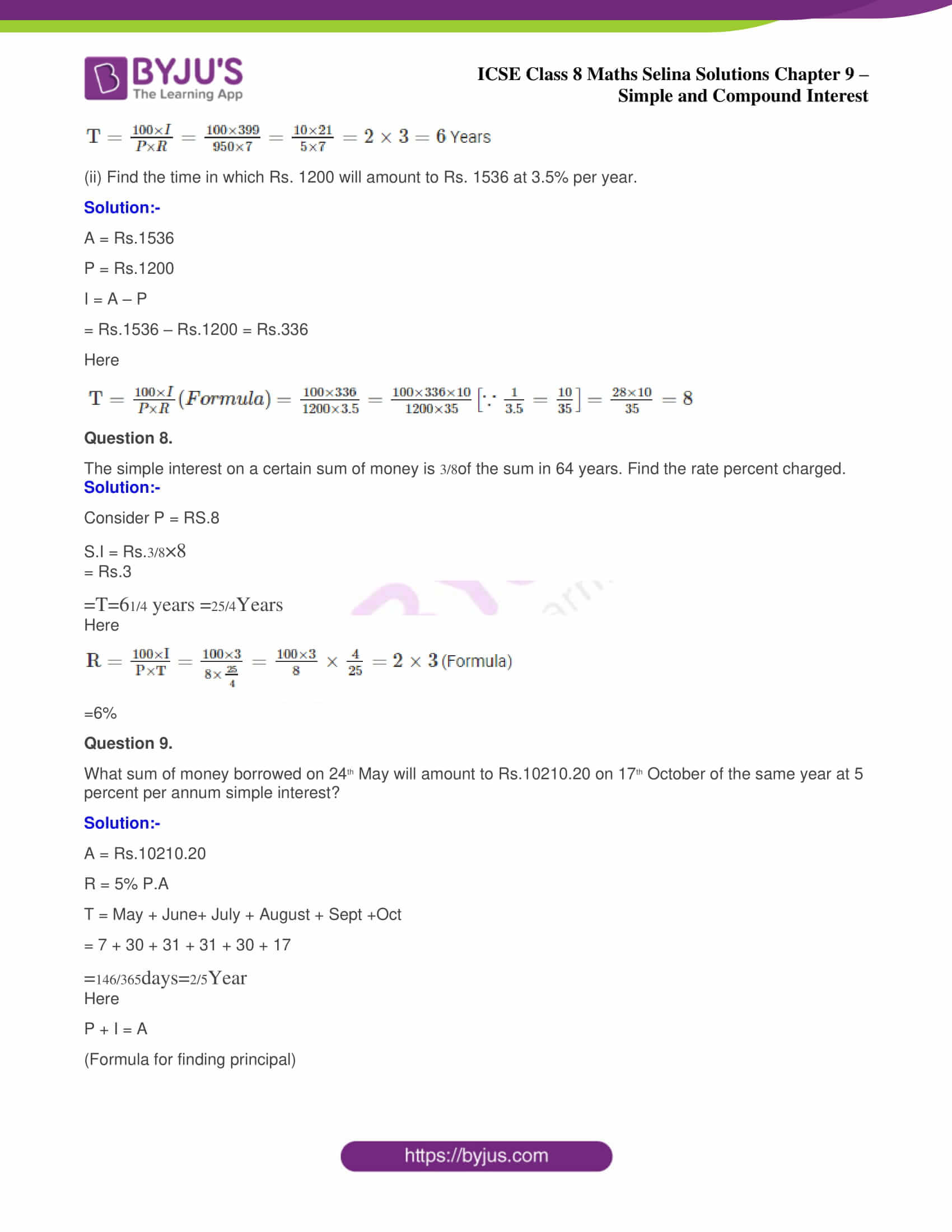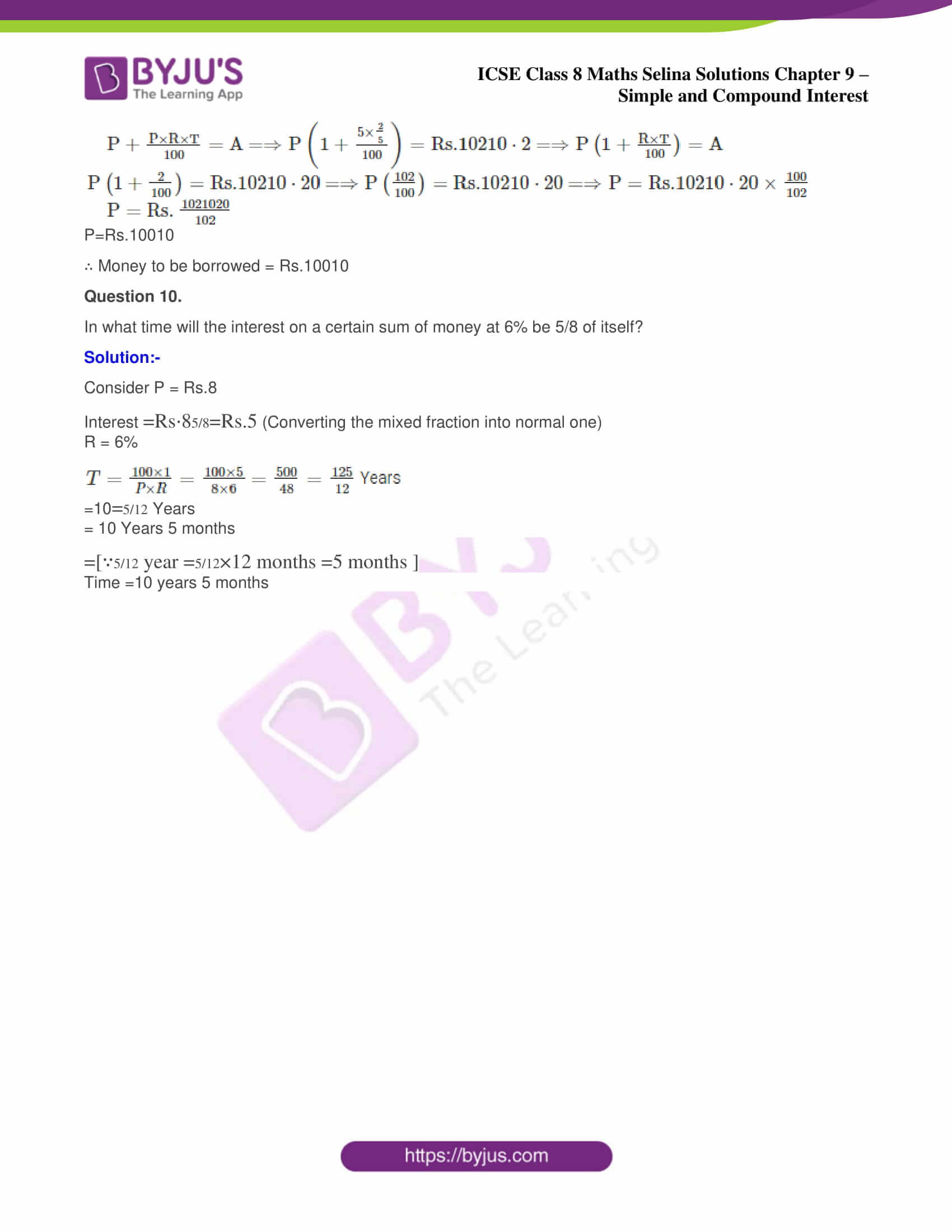ICSE Class 8 Maths Chapter 9 Simple and Compound Interest contains only 10 questions. The solutions of which are provided below. Also, you can access the solutions for Maths, Physics, Chemistry and Biology subjects at one place by visiting ICSE Class 8 Selina Solutions.

### ICSE Class 8 Maths Selina Solutions Chapter 9 Simple and Compound Interest – Exercise 9 Simple and Compound Interest (A)

Find the interest and the amount on:

(i) ₹ 750 in 3 years 4 months at 10% per annum.

Solution:-

Given P = ₹ 750

Time (T)

$$\begin{array}{l}=3 \frac{4}{12}=3 \frac{1}{3}=\frac{10}{3}\end{array}$$
= years

Rate (R)=10%

Interest (I)

$$\begin{array}{l}=\frac{P R T}{100}=\frac{750 \times 10 \times \frac{10}{3}}{100}\end{array}$$
$$\begin{array}{l}=\frac{250 \times 10 \times 10}{100}=250\end{array}$$

(ii) ₹ 5000 at 8% per year from 23rd December 2011 to 29th July 2012.

Solution:-

Principal (P) = ₹ 5000

Rate (R) = 8% p.a

Time (T) = 23 December 2011 to 29 July 2012

Dec. Jan. Feb. March April May June July

8 31 29 31 30 31 30 29

Total 219 days

$$\begin{array}{l}=\frac{219}{365}\end{array}$$
years

$$\begin{array}{l}=∴ \text { Interest }=\frac{P R T}{100}=\frac{5000 \times 8 \times 219}{100 \times 365}\end{array}$$
$$\begin{array}{l}=10 \times 8 \times 3=240\end{array}$$

∴Amount = P + I = ₹ 5000 + 240 = ₹ 5240

(iii) ₹ 2,600 in 2 years 3months at 1% per month.

Solution:-

Here p = ₹ 2600

Time (T) = 2 Years 3 month = 27 months

Rate (R) = 1 per month

$$\begin{array}{l}=∴ \text { Interest }=\frac{P \times T \times R}{100}=\frac{2600 \times 27 \times 1}{100}\end{array}$$
$$\begin{array}{l}=26 \times 27=\mathrm{Rs} .702\end{array}$$

Amount = (2600+702) = Rs.3302

(iv) ₹ 4,000 in

$$\begin{array}{l}=1 \frac{1}{3} \end{array}$$
years at 2 paise per rupee per month.

Solution:-

Here P=Rs.4.000, Time (T) =1

$$\begin{array}{l}=\frac{1}{3}\end{array}$$
year

= 1 year +

$$\begin{array}{l}=\frac{12}{3}\end{array}$$
months = 16 months

Rate (R) = 2 paise per rupee per month = 2% per month

$$\begin{array}{l}=\text { Interest }(I)=\frac{\mathrm{P} \times \mathrm{T} \times \mathrm{R}}{100}=\frac{4,000 \times 2 \times 16}{100}=40 \times 32=\mathrm{Rs} .1280\end{array}$$

Amount (A) =P+1=Rs.4000+Rs.1280 = Rs. 5280

Question 2

Rohit borrowed Rs. 24,000 at 7.5percent per year. How much money will he pay at the end of 4th years to clear his debt?

Solution:-

Principal (P) = Rs.24000

Rate (R) = 7.5% P.A.

Time (T) = 4 Years

S .I .

$$\begin{array}{l}=\frac{P \times T \times R}{100}\end{array}$$
$$\begin{array}{l}=\mathrm{Rs} . \frac{24,000 \times 4 \times 7.5}{100}\end{array}$$
$$\begin{array}{l}=\text { Rs. } 240 \times 4 \times 7.5\end{array}$$
$$\begin{array}{l}=240 \times 30=\mathrm{Rs} .7200\end{array}$$

Amount needed to clear the debt at the end of 4th year

= Rs.24000 + Rs.7200 = Rs.3,1200

Question 3.

The interest on a certain sum of money is Rs. 1,480 in 2 years and at 10 per cent per year. Find the sum of money.

Solution:-

Consider P = Rs. X

Time (T) = 2 Years

Rate (R) = 0%

$$\begin{array}{l}=∴ \text { Interest }=\frac{\mathrm{P} \times \mathrm{T} \times \mathrm{R}}{100}=\frac{x \times 10 \times 2}{100}=\frac{x}{5}\end{array}$$
$$\begin{array}{l}=\frac{x}{5}={Rs} .1480\end{array}$$
(Given)

$$\begin{array}{l}=∴ x=1480 \times 5={Rs} .7400 \end{array}$$

Therefore, the money Rs.7400

Question 4.

On what principal will the simple interest be Rs. 7,008 in 6 years 3 months at 5% per year?

Solution:-

Consider principal = Rs.P

Time (T) = 6 Years 3 month

$$\begin{array}{l}= 6 \mathrm{Year}+\frac{3}{12}\end{array}$$

Year

$$\begin{array}{l}=\frac{75}{12}=\frac{25}{4} \text { year }=6 \frac{1}{4} \end{array}$$
years

Rate(R)=5%

Simple interest =Rs.7,008

Here

Simple interest

$$\begin{array}{l}=\frac{\mathrm{P} \times \mathrm{T} \times \mathrm{R}}{100}\end{array}$$
$$\begin{array}{l}=7,008=\frac{\mathrm{P} \times \frac{25}{4} \times 5}{100} \Rightarrow \mathrm{P}=\frac{7008 \times 100 \times 4}{25 \times 5}\end{array}$$
$$\begin{array}{l}=\frac{7008 \times 16}{5}=\frac{112128}{5}={Rs} .22425 .60\end{array}$$

Question 5.

Find the principal which will amount to Rs. 4,000 in 4 years at 6.25% per annum.

Solution:-

Consider Principal = Rs P, Time (T) = 4 Years

Rate

$$\begin{array}{l}=6 \frac{1}{4}=\frac{25}{4} \% \end{array}$$

Simple Interest

$$\begin{array}{l}=\frac{\mathrm{P} \times \mathrm{T} \times \mathrm{R}}{100}=\frac{\mathrm{P} \times \frac{25}{4} \times 4}{100}=\frac{\mathrm{P}}{4}\end{array}$$
$$\begin{array}{l}=∴ \text { Amount }=P+\frac{P}{4}=\frac{5 P}{4} \end{array}$$
$$\begin{array}{l}=\frac{5 \mathrm{P}}{4}=4000\end{array}$$

5P=4×4000

P=Rs.3200

Therefore, Principal = Rs.3200

Question 6.

(i) At what rate per cent per annum will Rs. 630 produce an interest of Rs. 126 in 4 years?

Solution:-

P=Rs.630, I=Rs.126,T=4

$$\begin{array}{l}=R=\frac{100 \times I}{P \times T}=\frac{100 \times 126}{630 \times 4}=\frac{100}{20}=5 \%\end{array}$$

(ii) At what rate per cent per year will a sum double itself in

$$\begin{array}{l}=6 \frac{1}{4}\end{array}$$
years?

Solution:-

Let P = Rs.100

Amount = 2 × Rs.100 = Rs.200

Interest = A-P

= Rs.200 – Rs.100 = Rs.100

$$\begin{array}{l}\mathrm{T}=6 \frac{1}{4} \text { years }=\frac{25}{4}\end{array}$$
years

$$\begin{array}{l}\mathrm{R}=\frac{100 \times I}{\mathrm{P} \times \mathrm{T}}=\frac{100 \times 100}{100 \times \frac{25}{4}} \%=\frac{100 \times 100}{100} \times \frac{4}{25}=16 \%\end{array}$$

Question 7.

(i) In how many years will Rs. 950 produce Rs. 399 as simple interest at 7%?

Solution:-

P = Rs.950

S.I = Rs.399

R = 7%

Here

$$\begin{array}{l}\mathrm{T}=\frac{100 \times I}{P \times R}=\frac{100 \times 399}{950 \times 7}\end{array}$$
$$\begin{array}{l}\frac{10 \times 21}{5 \times 7}=2 \times 3=6\end{array}$$
Years

(ii) Find the time in which Rs. 1200 will amount to Rs. 1536 at 3.5% per year.

Solution:-

A = Rs.1536

P = Rs.1200

I = A – P

= Rs.1536 – Rs.1200 = Rs.336

Here

$$\begin{array}{l}\mathrm{T}=\frac{100 \times I}{P \times R} (Formula) \end{array}$$
$$\begin{array}{l}=\frac{100 \times 336}{1200 \times 3.5}=\frac{100 \times 336 \times 10}{1200 \times 35}\left[∵ \frac{1}{3.5}=\frac{10}{35}\right] \end{array}$$
$$\begin{array}{l}=\frac{28 \times 10}{35}=8\end{array}$$

Question 8.

The simple interest on a certain sum of money is

$$\begin{array}{l}\frac{3}{8} \end{array}$$
of the sum in 6
$$\begin{array}{l}\frac{1}{4} \end{array}$$
years. Find the rate percent charged.

Solution:-

Consider P = RS.8

S.I = Rs.

$$\begin{array}{l}=\frac{3}{8} \times 8\end{array}$$

= Rs.3

$$\begin{array}{l}\mathrm{T}=6 \frac{1}{4} \text { years }=\frac{25}{4} \mathrm{Years}\end{array}$$

Here

$$\begin{array}{l}\mathrm{R}=\frac{100 \times \mathrm{I}}{\mathrm{P} \times \mathrm{T}}\end{array}$$
$$\begin{array}{l}=\frac{100 \times 3}{8 \times \frac{25}{4}}=\frac{100 \times 3}{8} \times \frac{4}{25}=2 \times 3 \end{array}$$
(Formula)

=6%

Question 9.

What sum of money borrowed on 24th May will amount to Rs.10210.20 on 17th October of the same year at 5 percent per annum simple interest?

Solution:-

A = Rs.10210.20

R = 5% P.A

T = May + June+ July + August + Sept +Oct

= 7 + 30 + 31 + 31 + 30 + 17

$$\begin{array}{l}=\frac{146}{365} \mathrm{days}=\frac{2}{5} \mathrm{Year}\end{array}$$

Here

P + I = A

(Formula for finding principal)

$$\begin{array}{l}=\Rightarrow \quad \mathrm{P}+\frac{\mathrm{P} \times \mathrm{R} \times \mathrm{T}}{100}=\mathrm{A}\end{array}$$

$$\begin{array}{l}=\Rightarrow \mathrm{P}\left(1+\frac{5 \times \frac{2}{5}}{100}\right)=\mathrm{Rs} .10210 \cdot 2\end{array}$$

$$\begin{array}{l}=\Rightarrow \mathrm{P}\left(1+\frac{\mathrm{R} \times \mathrm{T}}{100}\right)=\mathrm{A}\end{array}$$

$$\begin{array}{l}=\Rightarrow \mathrm{P}\left(1+\frac{2}{100}\right)=\mathrm{Rs} .10210 \cdot 20\end{array}$$

$$\begin{array}{l}=\Rightarrow \mathrm{P}\left(\frac{102}{100}\right)=\mathrm{Rs} .10210 \cdot 20\end{array}$$

$$\begin{array}{l}=\Rightarrow \mathrm{P}=\mathrm{Rs} .10210 \cdot 20 \times \frac{100}{102}\end{array}$$

$$\begin{array}{l}=\Rightarrow \quad \mathrm{P}=\mathrm{Rs}. \frac{1021020}{102}\end{array}$$

P=Rs.10010

Money to be borrowed = Rs.10010

Question 10.

In what time will the interest on a certain sum of money at 6% be 5/8 of itself?

Solution:-

Consider P = Rs.8

Interest

$$\begin{array}{l}=\mathrm{Rs} \cdot 8 \frac{5}{8}=\mathrm{Rs} .5\end{array}$$
(Converting the mixed fraction into normal one)

R = 6%

$$\begin{array}{l}=T=\frac{100 \times 1}{P \times R}\end{array}$$
$$\begin{array}{l}=\frac{100 \times 5}{8 \times 6}\end{array}$$
$$\begin{array}{l}=\frac{500}{48}=\frac{125}{12}\end{array}$$
Years

=10

$$\begin{array}{l}= \frac{5}{12}\end{array}$$
Years

= 10 Years 5 months

$$\begin{array}{l}=\left[∵ \frac{5}{12} \text { year }=\frac{5}{12} \times 12 \text { months }=5 \text { months }\right] \end{array}$$

Time =10 years 5 months

To get the Selina Solutions for the chapters of class 8 Maths, visit the ICSE Class 8 Maths Selina Solutions page.

### ICSE Class 8 Maths Selina Solutions Chapter 9 – Simple and Compound Interest

In the earlier classes, you have learned about the simple interest. In this chapter, you will get introduced with the concept of compound interest. Also, you will know how the formula of Compound Interest is deduced. The difference between CI and SI is beautifully explained with the examples and also some of the problems provided in the exercises are based on it. So go through them and in case you get stuck solving any problem them refer to the solution pdf.

We hope this information on “ICSE Class 8 Maths Selina Solutions Chapter 9 Simple and Compound Interest” is useful for students. Keep learning and stay tuned for further updates on ICSE and other competitive exams. To access interactive Maths and Science Videos download BYJU’S App and subscribe to YouTube Channel.